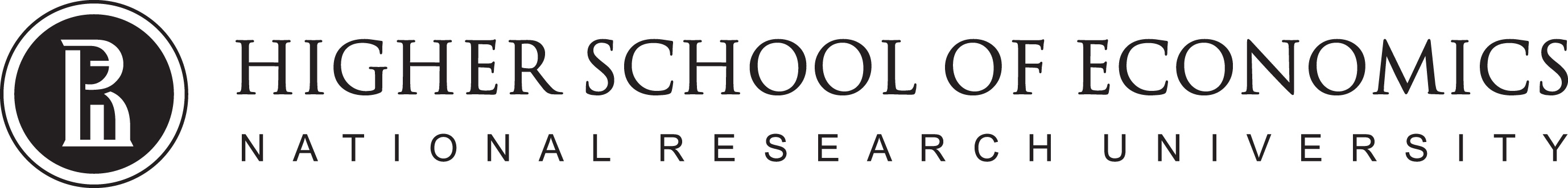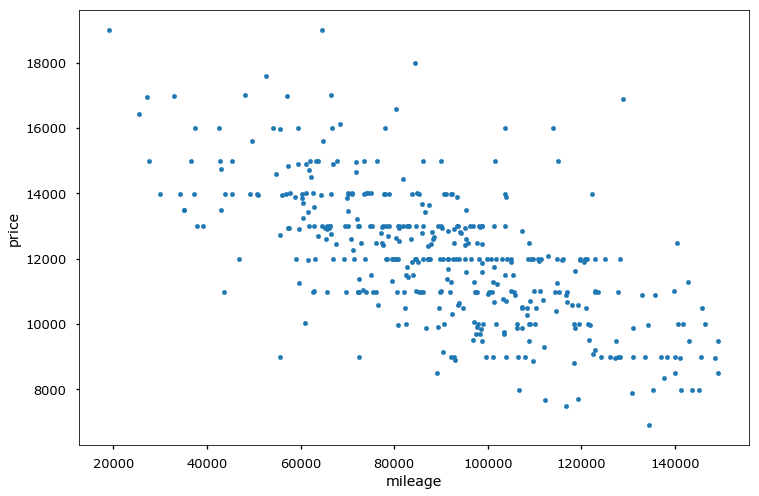# Data Analysis

## Linear classification. Logistic Regression1

1. Some materials are taken from machine learning course of Victor Kitov

## Wisdom of the day¶

### Overfitting = Death¶# Let's recall previous lecture¶

• Linear regression
• linear dependence between target features and predictors
$$f(x_{n}, \beta) = \hat{y}_{n} = \beta_0 + \beta_1x_{n}^1 + \dots$$
• Optimize Ordinary Least Squares
• Solution can be found
• analytically
• with gradient descent
In :
df_auto.plot(x='mileage', y='price', kind='scatter')

Out:
<matplotlib.axes._subplots.AxesSubplot at 0x1177e12e8>In :
from sklearn.linear_model import LinearRegression
X = df_auto.loc[:,['mileage']].values
y = df_auto.loc[:, 'price'].values

model = LinearRegression()
model.fit(X, y)
print('price = {:.2f} {:.2f}*mileage'.format(model.intercept_, model.coef_))

price = 16762.02 -0.05*mileage

In :
df_auto.loc[:, 'kilometerage'] = df_auto.loc[:,'mileage'] * 1.60934
X = df_auto.loc[:,['mileage', 'kilometerage']].values
y = df_auto.loc[:, 'price'].values

model = LinearRegression()
model.fit(X, y)
print('price = {:.2f} {:.2f}*mileage {:.2f}*kilometerage'.format(model.intercept_, *model.coef_))

price = 16762.02 -0.01*mileage -0.02*kilometerage


# Regularization & restrictions¶

## Intuition¶[Andrew's Ng Machine Learning Class - Stanford]

## Regularization¶

• Insert regularizer $R(\beta)$ for $\beta$ to be small: $$\sum_{n=1}^{N}\left(x_{n}^{T}\beta-y_{n}\right)^{2}+\lambda R(\beta)\to\min_{\beta}$$
• $\lambda>0$ - hyperparameter.
• $R(\beta)$ penalizes complexity of models. $$\begin{array}{ll} R(\beta)=||\beta||_{1} & \mbox{Lasso regression}\\ R(\beta)=||\beta||_{2}^{2} & \text{Ridge regression} \end{array}$$

• Not only accuracy matters for the solution but also model simplicity!